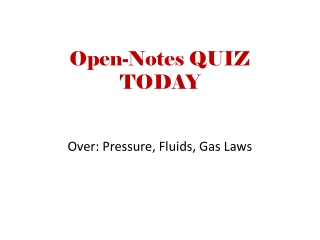DownloadDownload PresentationOpen-Notes QUIZ TODAY

# Open-Notes QUIZ TODAY

Télécharger la présentation## Open-Notes QUIZ TODAY

- - - - - - - - - - - - - - - - - - - - - - - - - - - E N D - - - - - - - - - - - - - - - - - - - - - - - - - - -
##### Presentation Transcript

1. Open-Notes QUIZ TODAY Over: Pressure, Fluids, Gas Laws

2. Gas Laws Bellwork – When gas in a hot-air balloon is heated, does it become more dense or less dense ( why does the balloon rise? )

3. Gas Laws Bellwork – When gas in a hot-air balloon is heated, does it become more dense or less dense ( why does the balloon rise? ) The gas is less dense / more spread out Particles are further apart

4. Gas Laws • How did we define a LAW?

5. Gas Laws • How did we define a LAW? • PREDICTS some action or behavior • Gas Laws predict gas behavior in specific conditions • CONDITIONS are Pressure, Temperature, Volume

6. Temperature • When we deal with temperature in these gas laws, absolute temperature is used • Kelvin scale • Through examples, you will see why we have the Kelvin scale

7. Bellwork – 02/05/15 • With constant temperature, do you think pressure and volume are directly or inversely proportional? • inversely • We will use a simulation

8. Boyle’s Law • Product of pressure and volume is constant • Temperature is held constant • Pressure and Volume are INVERSELY Proportional • P1V1 = P2V2 = CONSTANT • “1” indicates initial conditions or a “before” • “2” indicates final conditions or an “after”

9. Gay-Lussac’s Law

10. Charles’s Law

11. Go back to Labtops –TAKE NOTES • Again at the PhET site, search for “Gas Properties” simulation • RUN simulation • Add gas ( choose light species ) – set value to 1 • See that this number tells you # of particles • Increase gas value to 150 (USE PUMP , then adjust)

12. Example of Boyle’s Law • If a gas has a volume of 325 mL at a pressure of 478 mmHg, what will the pressure be at a volume of 416 mL? • P1V1 = P2V2 = CONSTANT

13. Example of Boyle’s Law • P1V1 = P2V2 = CONSTANT • ( 478 ) * ( 325 ) = 155350 ( our constant ) • 155350 = P2V2 = P2 * ( 416 ) • P2 = 155350 / 416 = 373 mmHg

14. Using Boyle’s Law • From this example, how are pressure and volume related? • As Pressure increased, volume decreased • The opposite can happen ( vol increase, pressure decrease ) • This is what meant by INVERSELY proportional